# Central Limit Theorem Section 5 5 M A

• Slides: 20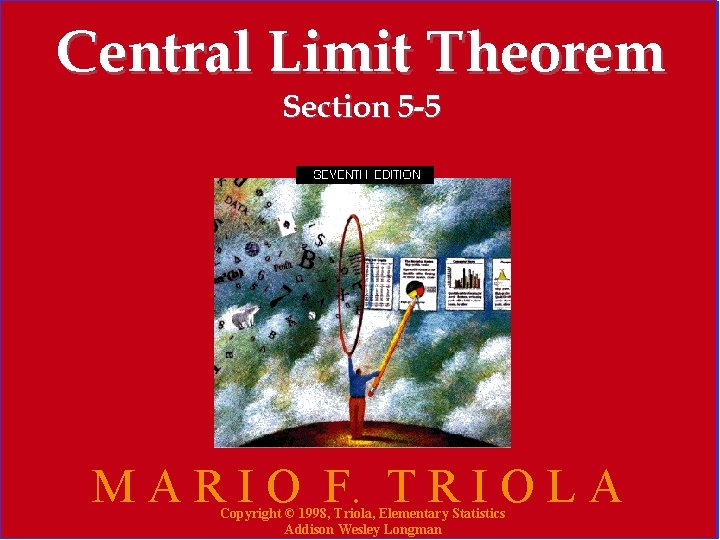Central Limit Theorem Section 5 -5 M A R I O F. T R I O L A Copyright © 1998, Triola, Elementary Statistics Addison Wesley Longman 1As the sample size increases the distribution of sample means will approach a normal distribution. Copyright © 1998, Triola, Elementary Statistics Addison Wesley Longman 2Distribution of 200 digits From Social Security Numbers Frequency (Last 4 digits from 50 students) 20 10 0 0 1 2 3 4 5 6 7 8 9 Distribution of 200 digits Figure 5 -19 Copyright © 1998, Triola, Elementary Statistics Addison Wesley Longman 3Table 5 -1 x SSN digits 1 5 9 4 7 9 5 7 8 3 8 1 3 2 7 1 3 83 2 6 2 2 5 0 2 7 8 5 7 7 3 4 4 4 5 1 3 6 6 3 8 2 3 6 1 5 3 42 6 7 3 3 8 3 7 6 4 6 8 5 5 2 6 4 9 4. 75 4. 25 8. 25 3. 25 5. 00 3. 50 5. 25 4. 75 5. 00 2 6 1 9 5 7 8 6 4 0 7 4. 00 5. 25 4. 50 4. 75 3. 75 5. 25 3. 75 4. 50 6. 00 Copyright © 1998, Triola, Elementary Statistics Addison Wesley Longman 4Frequency Distribution of 50 Sample Means for Students 15 10 5 0 0 1 2 3 4 5 6 7 8 9 Distribution of 50 Sample Means Figure 5 -20 Copyright © 1998, Triola, Elementary Statistics Addison Wesley Longman 5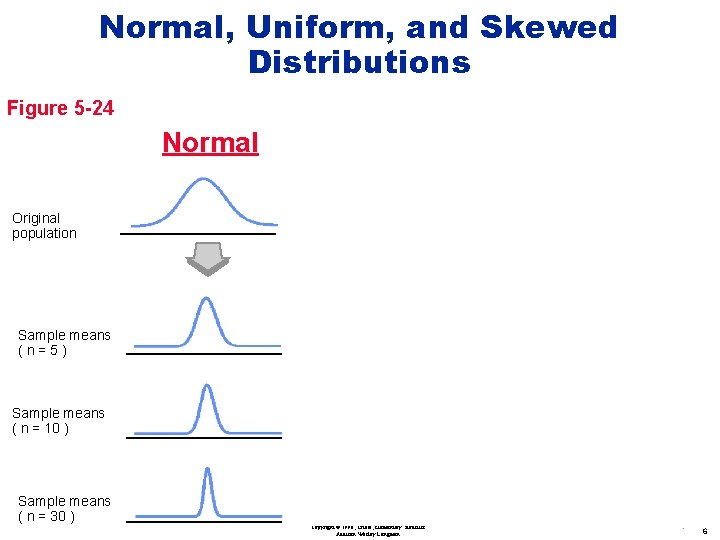Normal, Uniform, and Skewed Distributions Figure 5 -24 Normal Uniform Skewed Original population Sample means (n=5) Sample means ( n = 10 ) Sample means ( n = 30 ) Copyright © 1998, Triola, Elementary Statistics Addison Wesley Longman 6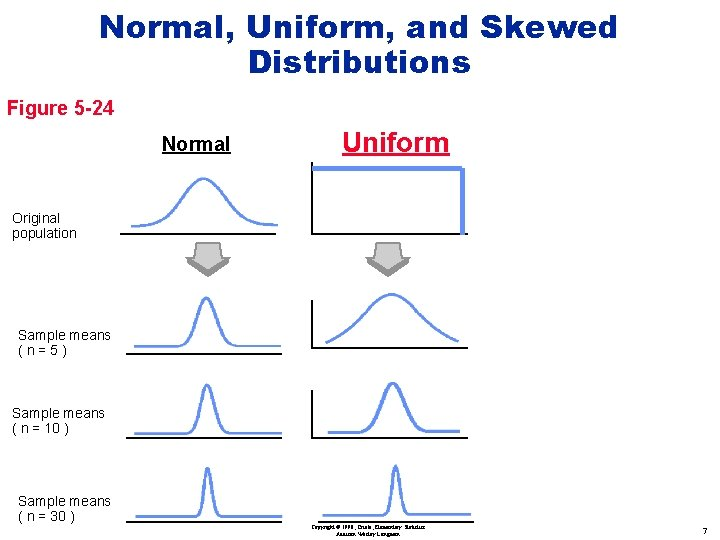Normal, Uniform, and Skewed Distributions Figure 5 -24 Normal Uniform Original population Sample means (n=5) Sample means ( n = 10 ) Sample means ( n = 30 ) Copyright © 1998, Triola, Elementary Statistics Addison Wesley Longman 7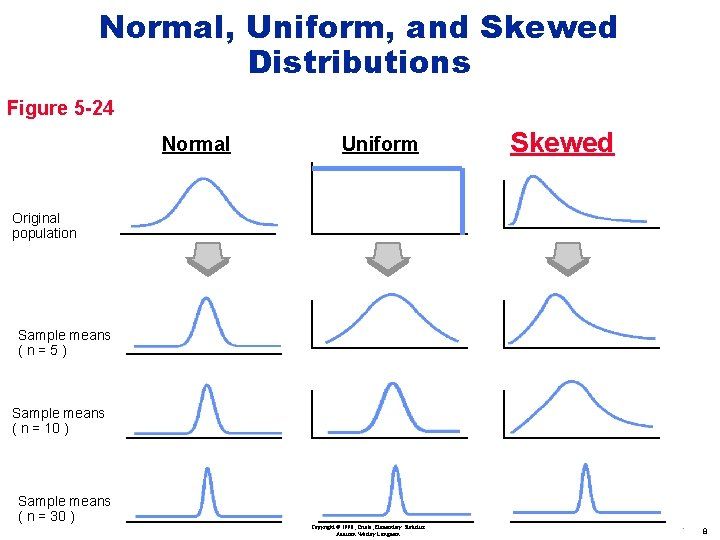Normal, Uniform, and Skewed Distributions Figure 5 -24 Normal Uniform Skewed Original population Sample means (n=5) Sample means ( n = 10 ) Sample means ( n = 30 ) Copyright © 1998, Triola, Elementary Statistics Addison Wesley Longman 8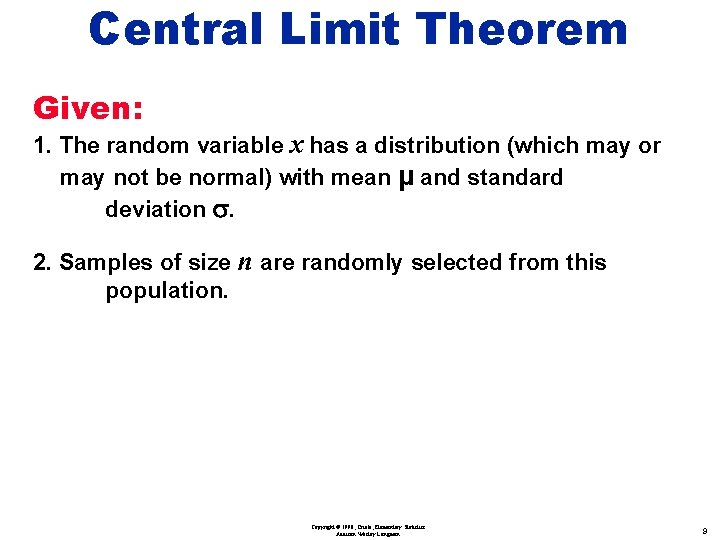Central Limit Theorem Given: 1. The random variable x has a distribution (which may or may not be normal) with mean µ and standard deviation s. 2. Samples of size population. n are randomly selected from this Copyright © 1998, Triola, Elementary Statistics Addison Wesley Longman 9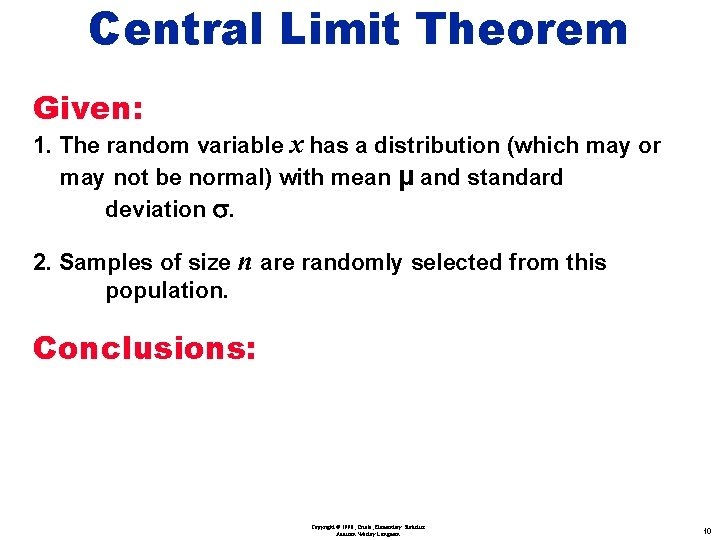Central Limit Theorem Given: 1. The random variable x has a distribution (which may or may not be normal) with mean µ and standard deviation s. 2. Samples of size population. n are randomly selected from this Conclusions: Copyright © 1998, Triola, Elementary Statistics Addison Wesley Longman 10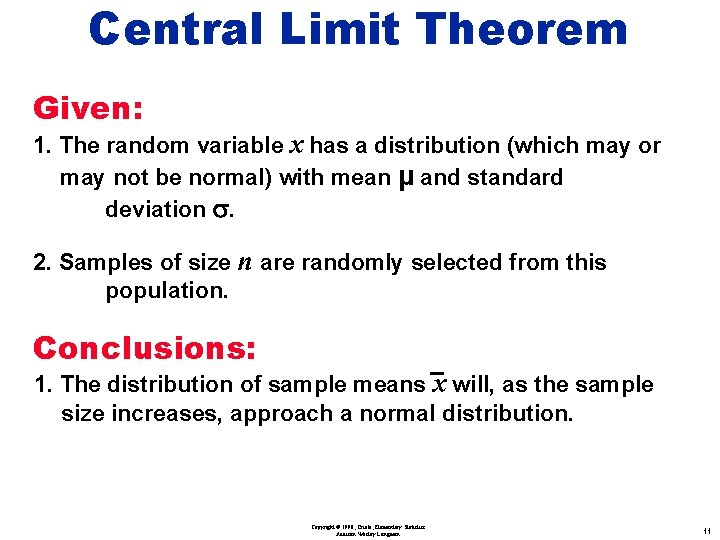Central Limit Theorem Given: 1. The random variable x has a distribution (which may or may not be normal) with mean µ and standard deviation s. 2. Samples of size population. n are randomly selected from this Conclusions: 1. The distribution of sample means x will, as the sample size increases, approach a normal distribution. Copyright © 1998, Triola, Elementary Statistics Addison Wesley Longman 11Central Limit Theorem Given: 1. The random variable x has a distribution (which may or may not be normal) with mean µ and standard deviation s. 2. Samples of size population. n are randomly selected from this Conclusions: 1. The distribution of sample means x will, as the sample size increases, approach a normal distribution. 2. The mean of the sample means will be the population mean µ. s 3. The standard deviation of the sample means will be — n Copyright © 1998, Triola, Elementary Statistics Addison Wesley Longman 12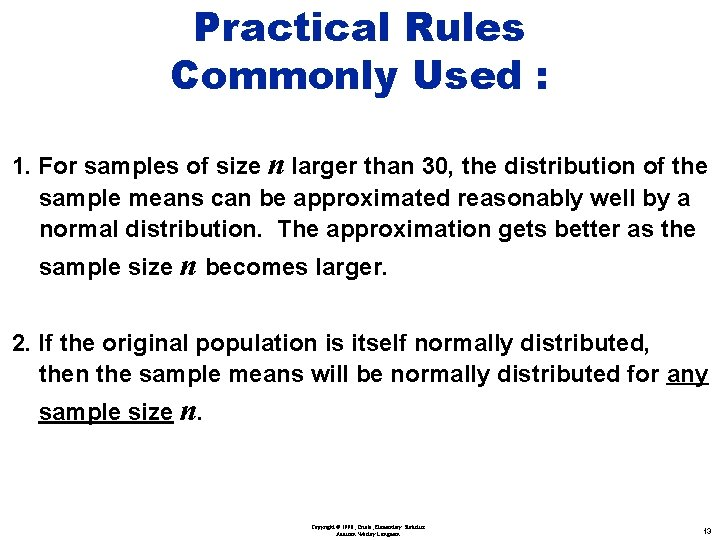Practical Rules Commonly Used : 1. For samples of size n larger than 30, the distribution of the sample means can be approximated reasonably well by a normal distribution. The approximation gets better as the sample size n becomes larger. 2. If the original population is itself normally distributed, then the sample means will be normally distributed for any sample size n. Copyright © 1998, Triola, Elementary Statistics Addison Wesley Longman 13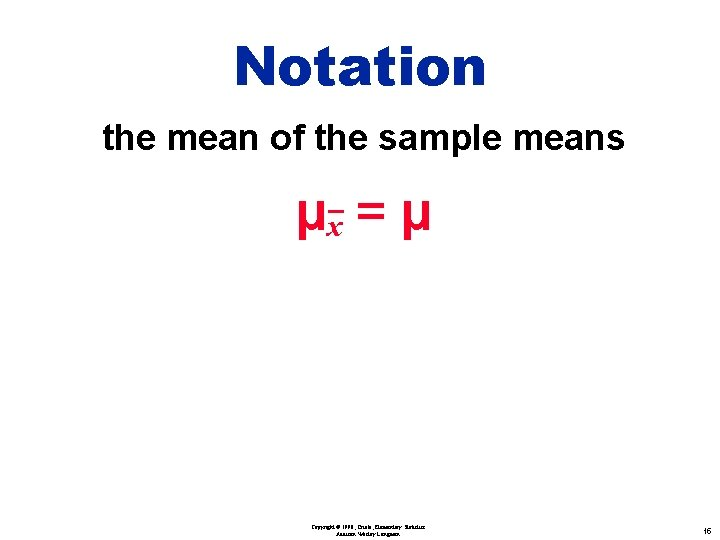Notation the mean of the sample means µx = µ the standard deviation of sample mean sx = s n (often called standard error of the mean) Copyright © 1998, Triola, Elementary Statistics Addison Wesley Longman 15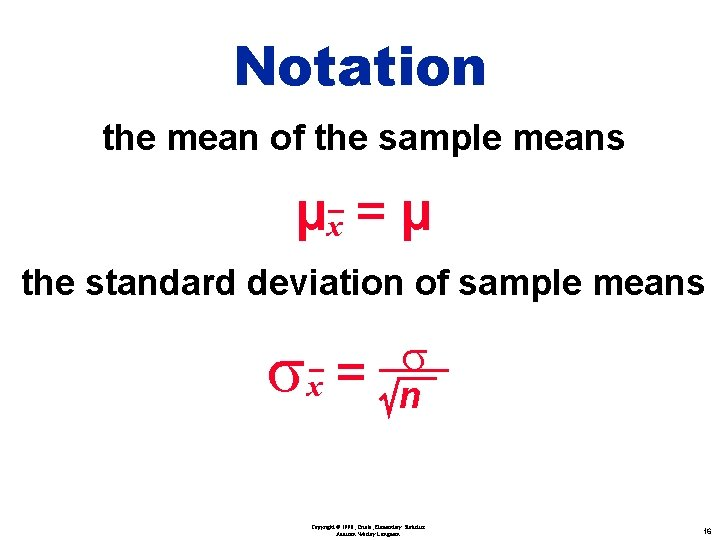Notation the mean of the sample means µx = µ the standard deviation of sample means sx = s n (often called standard error of the mean) Copyright © 1998, Triola, Elementary Statistics Addison Wesley Longman 16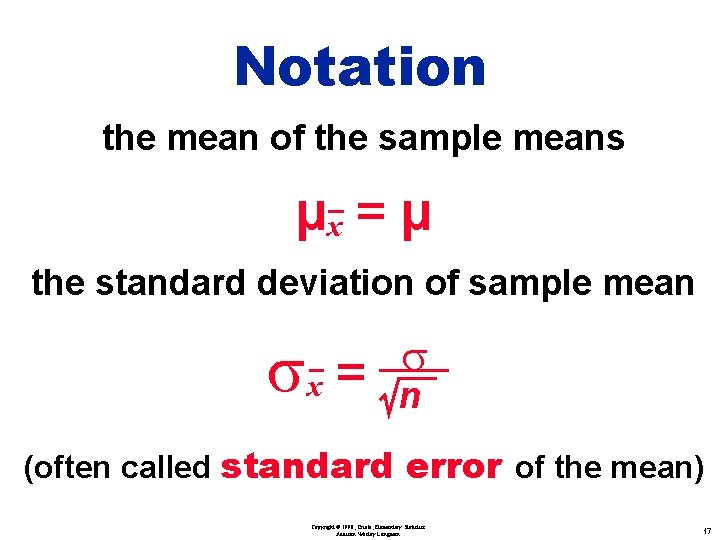Notation the mean of the sample means µx = µ the standard deviation of sample mean sx = s n (often called standard error of the mean) Copyright © 1998, Triola, Elementary Statistics Addison Wesley Longman 17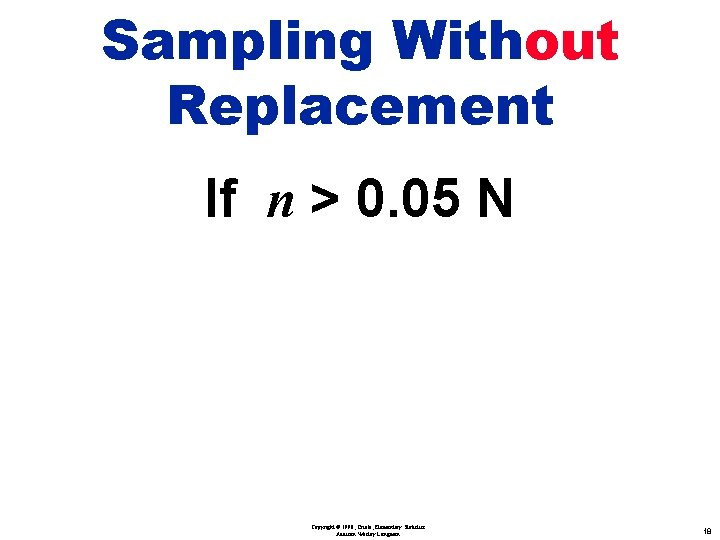Sampling Without Replacement If n > 0. 05 N Copyright © 1998, Triola, Elementary Statistics Addison Wesley Longman 18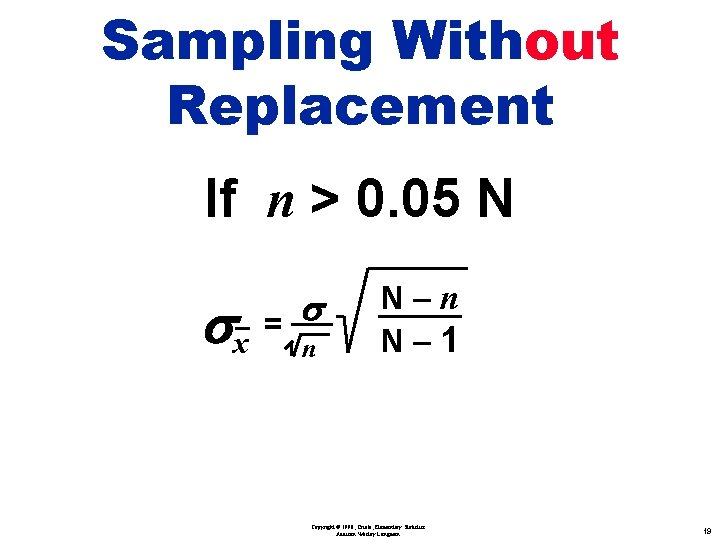Sampling Without Replacement If n > 0. 05 N s sx = n N– 1 Copyright © 1998, Triola, Elementary Statistics Addison Wesley Longman 19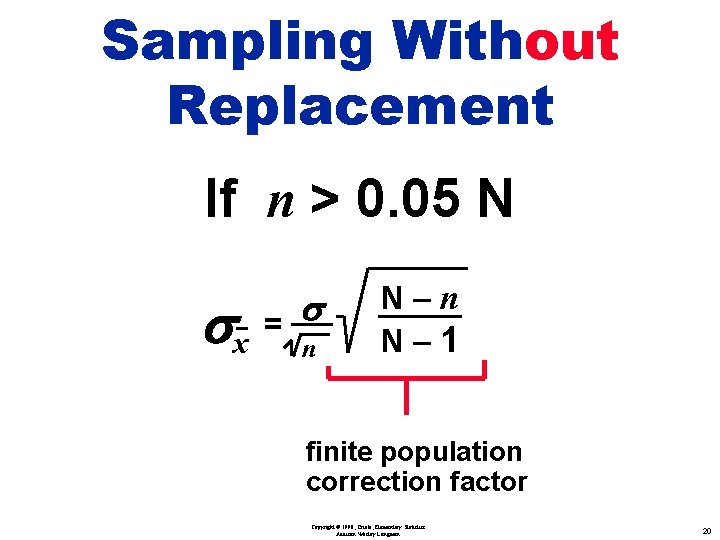Sampling Without Replacement If n > 0. 05 N s sx = n N– 1 finite population correction factor Copyright © 1998, Triola, Elementary Statistics Addison Wesley Longman 20